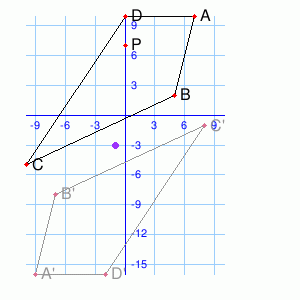# FORM TWO MATHEMATICS STUDY NOTES TOPIC 5-7.

0
347TOPIC 5: CONGRUENCE

Congruence
I’m
sure you have seen some of the figure which in one way or another one
of the shape can become another using turns, flip or slide. These shapes
are said to be Congruent. Study this notes carefully to know different
In
geometry two figures or objects are congruent if they have the same
shape and size, or if one has the shape and size as the mirror image of
the other.
If
one shape can become another using turns (rotation), flip (reflection),
and/ or slide (translation), then the shapes are Congruent. After any
of these transformations the shape must still have the same size,
perimeters, angles, areas and line lengths.
Note that; the two shapes need to be the size to be Congruent i.e. only rotation, reflection and/ or translation is needed.
Remember this:
• Two line segments are Congruent if they have the same length.
• Two angles are Congruent if they have the same measure.
• Two circles are Congruent if they have the same diameter.
Angles formed by the intersection of two straight lines
When
two straight lines intersect, they form four angles. Each opposite pair
are called vertical angles and they are congruent. Vertical angles are
also called opposite angles. See figure below for more understanding: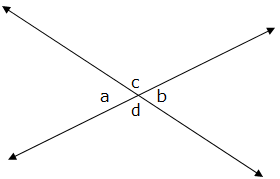Properties of vertical angles
• They are Congruent: vertical angles are always of equal measure i.e. a = b, and c = d.
• Sum of vertical angles (all four angles) is 3600 i.e. a + b + c + d =3600
• Sum of Adjacent angles (angles from each pair) is 1800 i.e. a + d =1800 ; a + c =1800 ; c + b =1800 ; b + d =1800.
Two
Triangles are Congruent if their corresponding sides are equal in
length and their corresponding angles are equal in size. The symbol for
congruent shapes is
The Conditions for Congruence of Triangles
Determine the conditions for congruence of triangles
The following are conditions for two Triangles to be Congruent:
• SSS
(side-Side-Side): if three pairs of sides of two Triangles are equal in
length, then the Triangles are Congruent. Consider example below
showing two Triangles with equal lengths of the corresponding sides.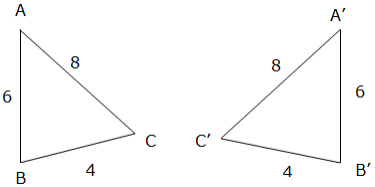Example 1
Prove that the two Triangles (ΔABC and ΔBCD) below are Congruent.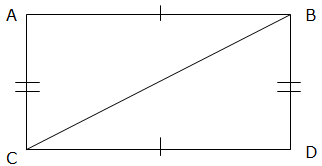Solution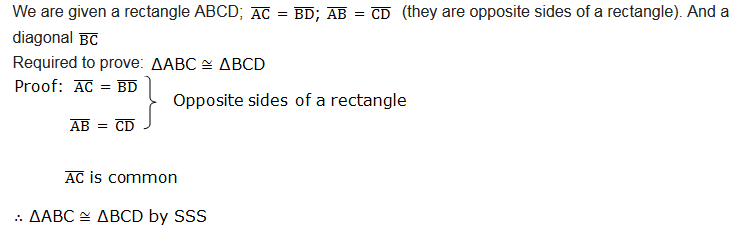Another condition;
• SAS
(Side-Angle-Side): This means that we have two Triangles where we know
two sides and the included angles are equal. For example;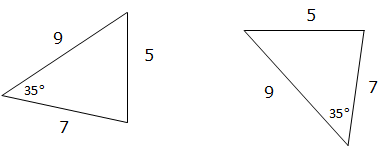If
the two sides and the included angle of one Triangle are equal to
corresponding sides and the included angle of the other Triangle, we say
that the two Triangles are Congruent.
• ASA
(Angle- Side-Angle): If two angles and the included side of one
Triangle are equal to the two angles and included side of another
Triangle we say that the two Triangles are congruence. For example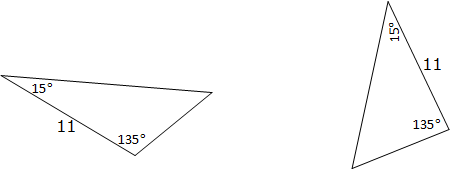AAS condition;
• AAS
(Angle-Angle-Side): If two angles and non included side of one triangle
are equal to the corresponding angles and non included side of the
other Triangle, then the two triangles are congruent. For example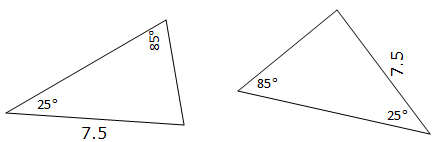• HL
(hypotenuse-Leg): This is applicable only to a right angled triangle.
The longest side of a right angled triangle is called hypotenuse and the
other two sides are legs.
It means we have two right angled triangles with:
• The same length of hypotenuse and
• The same length for one of the other two legs.
If
the hypotenuse and one leg of one right angled triangle are equal to a
corresponding hypotenuse and one leg of the other right angled triangle,
the two triangles are congruent. For exampleImportant
note: Do not use AAA (Angle-Angle-Angle). This means we are given all
three angles of a triangle but no sides. This is not enough information
to decide whether the two triangles are congruent or not because the
Triangles can have the same angles but different size. See an
illustration below: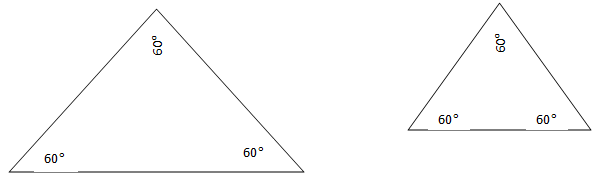The two triangles are not congruent.
Without knowing at least one side, we can’t be sure that the triangles are congruent.
Congruence of Triangle
Prove congruence of triangle
Example 2
Prove that the two Triangles (ΔABC and ΔBCD) below are Congruent.Solution;Theorems on Congruence of Triangles to Solve Related Problems
Apply theorems on congruence of triangles to solve related problems
Isosceles Triangle Theorem
The figure below illustrates an example of an isosceles triangle: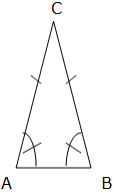An
isosceles triangle has two congruent sides (opposite sides) and two
congruent angles. The congruent angles are called base angles and the
other angle is called vertex angle. The angles A and B are base angles
and angle C is the vertex angle.
The base angle Theorem
If two sides of a triangle are congruent, then the angles opposite to these sides are congruent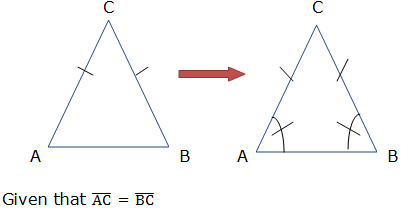Required to prove: angle A = angle B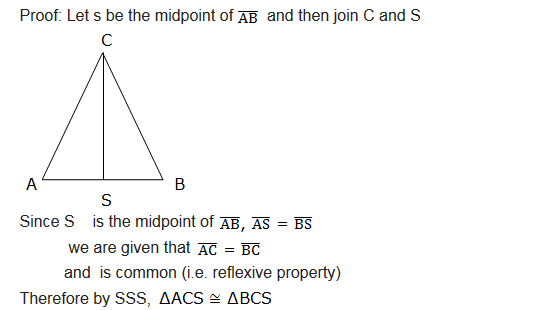Therefore, the base angles i.e. angle CAS and angle BCS are equal (by the definition of a congruence of triangles).
It states that, if two angles of a triangle are congruent, then sides opposite those angles are congruent.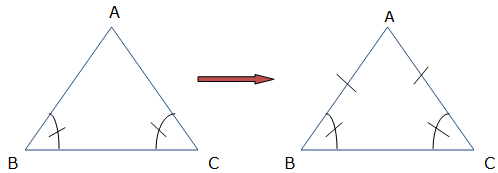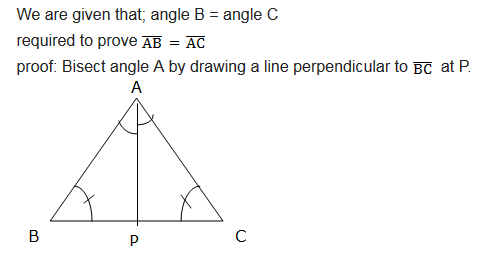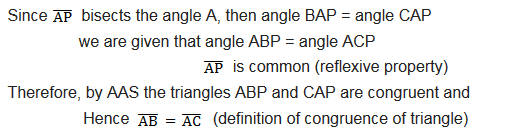Exercise 1
1.
In the isosceles triangle ABC, BA and BC are congruent. D and E are
points on AC such that AD is congruent to BD and BE is congruent to BC.
Show that the triangles ABD and CBE are congruent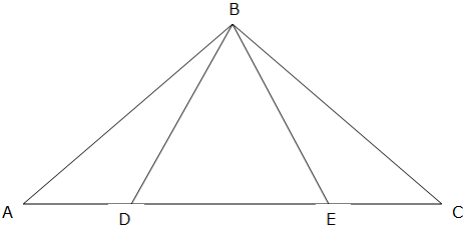2. ABCD is a parallelogram and BEFC is a square. Show that triangles ABE and DCF are congruent.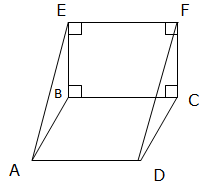3. Use the figure below to answer the following questions: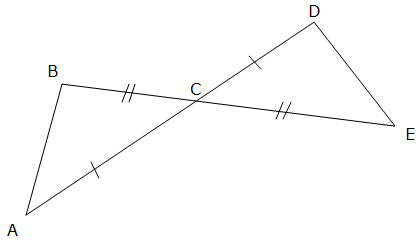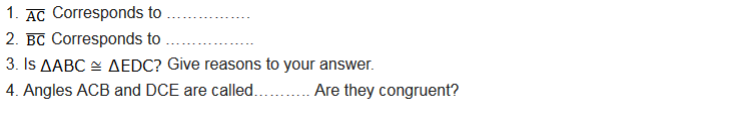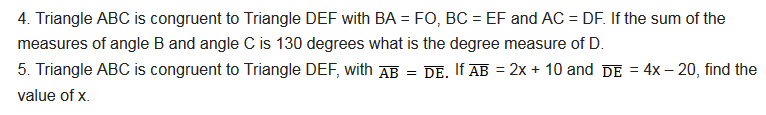TOPIC 7: GEOMETRIC AND TRANSFORMATIONS

Reflection
The Characteristics of Reflection in a Plane
Describe the characteristics of reflection in a plane
A
transformation in a plane is a mapping which moves an object from one
position to another within the plane. Think of a book being taken from
one comer of a table to another comer. Figures on a plane of paper can
also be shifted to a new position by a transformation. The new postion
after a transformation is called the image. Examples of transformations
are reflection, rotation, enlargement and translation.
Different Reflections by Drawings
Represent different reflections by drawings
A
reflection is a transformation which reflects all points of a plane in a
line called the mirror-line. The image in a mirror is as far behind the
mirror as the object is in front of the mirrorCharacteristics of Reflection
In
the diagram, APQR is mapped onto ΔP’Q’R’ under a reflection in the line
AB. If the paper is folded along the line AB, ΔPQR will fall in exactly
onto ΔPQR. The line AB is the mirror-line. which is the perpendicular
bisector of PP’, QQ’ and ΔPQR and ΔP’Q’R are congruent.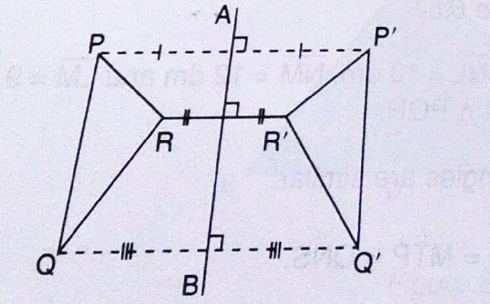Some characteristics observed under reflection are:
1. PP’ is perpendicular to AB, RR’ is perpendicular to AB and QQ is perpendicular to AB.
2. The image of any point on the Q’ mirror line is the point itself.
3. PP’ is parallel to RR’ and QQ’
Reflection in the Line y = x
The
line y = x makes an angle 45° with the x and y axes. It is the line of
symmetry for the angle YOX formed by the two axes. By using the
isosceles triangle properties, reflection of the point (1, 0) in the
line y = x will be (0, 1).
The
reflection of (0,2) in the liney = x will be (2,0). You notice that the
co-ordinates are exchanging positions. Generally, the reflection of the
point (a,b) in the line y = x is (b,a).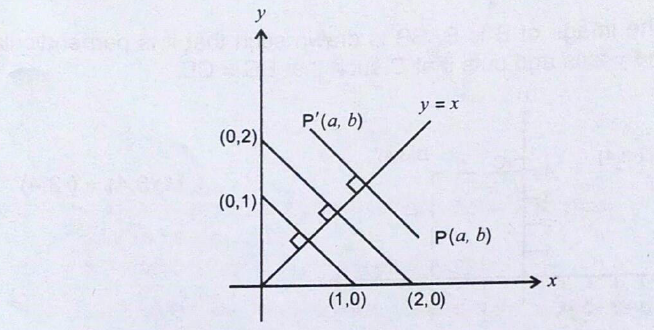The reflection of the point B(c,d) in the line y = -x is B’ (-d, -c)
Exercise 1
1. Find the image of the point D(4,2) under a reflection in the x-axis.
2. Find the image of the point P(-2,5) under a reflection in the x-axis.
3. Point Q(-4,3) is reflected in the y-axis. Find the coordinates of its image.
4. Point R(6,-5) is reflected in the y-axis. Find the co-ordinates of its image.
5. Reflect the point (1 ,2) in the line y = -x.
6. Reflect the point (5,3) in the line y = x.
7. Find the image of the point (1 ,2) after a reflection in the line y=x followed by another reflection in the line y = -x.
8. Find
the image of the point P(-2,1) in the line y = -x followed by another
reflection in the line x = 0 ketch the positions of the image P and the
point P, indicating clearly the lines involved.
9. Find the co-ordinates of the image of the point A(5,2) under a reflection in the line y = 0.
10. Find the coordinates of the image of the point under a reflection in the line x = 0.
11. The co-ordinates of the image of a point R reflected in the x axis is R(2, -9). Find the coordinates of R.
Combined Transformations
Draw combined transformations
Combined
Transformation means that two or more transformations will be Performed
on one object. For instance you could perform a reflection and then a
translation on the same point
Example 3
What type of transform takes ABCD to A’B’C’D’?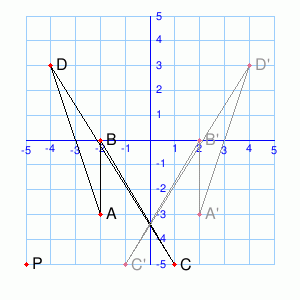Solution
The type of transform takes ABCD to A’B’C’D’ is Reflection
Simple Problems on Combined Transformations
Solve simple problems on combined transformations
Exercise 5
What type of transform takes ABCD to A’B’C’D’?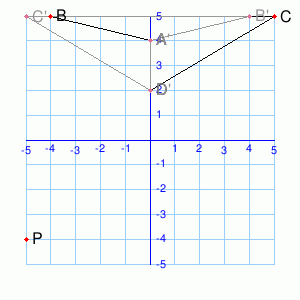The
transformation ABCD → A’B’C’D’ is a rotation around(-1, 2)by___°.Rotate
P around(-1, 2)by the same angle. (You may need to sketch things out on
paper.)P’ = (__,__)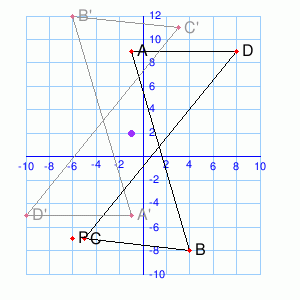The
transformation ABCD → A’B’C’D’ is a rotation around(-1, -3)by__°Rotate P
around(-1, -3)by the same angle. (You may need to sketch things out on
paper.)P’ = (__,__)